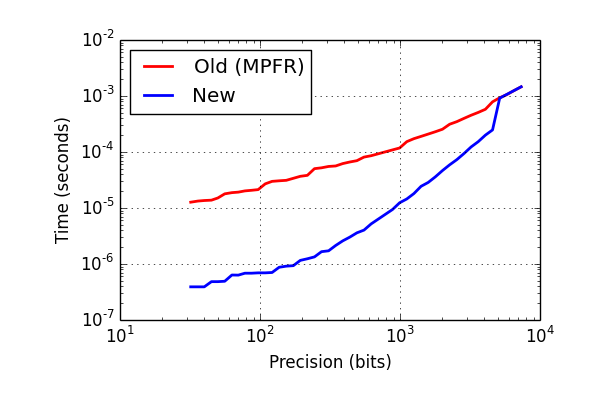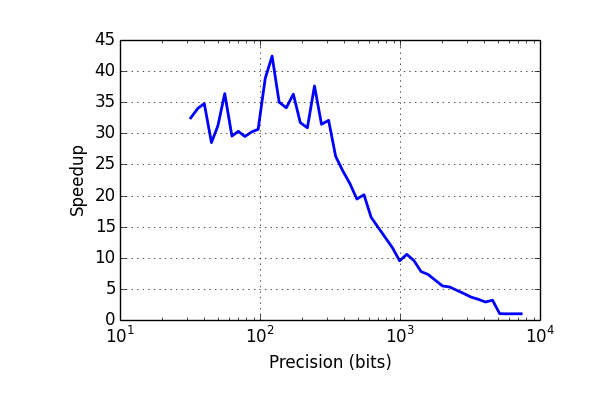# Thirty times faster arctangents

August 12, 2014

I have rewritten the arctangent function in Arb to improve speed at low-to-medium precision (up to about 1000 digits). At 10-100 digits, it is now 30 times faster than wrapping MPFR. The arctangent is a bottleneck when computing complex logarithms, so this should speed up lots of code.

Here are some benchmark results on an Intel Pentium T4400, for computing the arctangent of $x = \sqrt{3}/2$ (including the cost of error propagation). The times are in seconds, i.e. a 64-bit arctangent now just takes 600 nanoseconds.

Precision (bits) Old (MPFR) New Speedup
32 0.000012600 0.000000395 32x
64 0.000018800 0.000000600 31x
128 0.000030000 0.000000880 34x
256 0.000050800 0.000001750 29x
512 0.000076200 0.000003980 19x
1024 0.000139000 0.000013600 10x
2048 0.000283000 0.000050600 5.5x
4096 0.000677000 0.000205000 3.3x
8192 0.001880000 0.001890000 1x

In pictures:The new implementation is based on the following techniques:

• Table-based argument reduction is used to give rapid convergence of the Taylor series
• The Taylor series is evaluated using a fast version of the rectangular splitting scheme (avoiding divisions)
• To minimize overhead, everything is done using mpn functions

In the near future, I plan to do a similar rewrite of the other real elementary functions (exp, log, sin, cos). The algorithm is simple, but it took me several weeks to implement (the other functions should be slightly easier). Most of the difficulty comes from using mpn functions, in particular constructing an algorithm that takes advantage of the mpn layer as much as possible (e.g. doing a single mpn_addmul_1 whenever possible) while also being able to prove that no overflows are possible and that the error bounds are correct. This is mostly in my head and on scratch paper right now, but I will write more documentation later. I should point out that I have not tested it on 32-bit systems yet.

## Argument reduction

Starting with an input in the range $[0,1)$, one can repeatedly apply the formula $$\operatorname{atan}(x) = 2 \operatorname{atan}\left(\frac{x}{1+\sqrt{1+x^2}}\right)$$ which approximately halves $x$ each time. Applying this $r$ times makes $x$ smaller than $2^{-r}$, which means that only about $0.5 p / r$ terms of the Taylor series are needed for an accuracy of $p$ bits (the factor 0.5 comes from the fact that the even-index terms in the Taylor series vanish). This strategy mirrors computing the exponential function as $\exp(x) = \exp(x/2^r)^{2^r}$ and is quite effective. However, each halving is very expensive due to the square root and division. It is better to use $$\operatorname{atan}(x) = \operatorname{atan}(p/q) + \operatorname{atan}\left(\frac{qx-p}{px+q}\right)$$ where $p/q$ is a small rational number close to $x$. If we take $q = 2^r$ and store $\operatorname{atan}(p/q)$ for $p = 0, 1, \ldots, q-1$ in a lookup table, we get the equivalent of $r$ halvings almost for free, requiring just a single multiprecision division. The drawback is that we have to store a lookup table (the $\operatorname{atan}(p/q)$ values can be computed efficiently using binary splitting, but they still have to be cached to really make it worthwhile). A version of this algorithm is already used in mpmath.

## Taylor series

The Taylor series $\operatorname{atan}(x) \approx x - x^3/3 + x^5/5 + \ldots \pm x^{2N-1} / (2N-1)$ is evaluated using a version of Smith's "concurrent summation" method which requires $O(N^{1/2})$ multiprecision multiplications. The "rectangular splitting" version is used instead of the "modular splitting" originally proposed by Smith, as this allows using Horner's method for the outer polynomial evaluation which is slightly more efficient. These methods are discussed in the excellent book Modern Computer Arithmetic by Brent and Zimmermann.

I added one more very significant improvement: instead of doing a scalar division for each term, I collect denominators to replace divisions with scalar multiplications which can be done with single mpn_addmul_1 or mpn_submul_1 calls (also getting the additions nearly for free). Divisions are still required, but the number of divisions is greatly reduced, especially on 64-bit (for moderate $N$, the least common multiple of several consecutive denominators fits in a single limb).

## Lookup tables

At precisions up to just less than 512 bits, a 256-entry table is used, giving 8 halvings worth of argument reduction. At precisions up to slightly less than 4608 bits, a pair of 32-entry tables is used, giving 10 halvings worth of argument reduction (using a pair of tables costs an extra division). The total size of the tables in memory is 256 x 512 + 2 x 32 x 4608 bits = 52 KB. These sizes were chosen unscientifically to give good performance at 10, 100 and 1000 digits as well as 64, 256 and 4096 bits of precision (typical precisions for benchmarking!). One can get slightly better speed by doubling the size of the table, or one can reduce the table to half the size at the cost of a slight reduction in speed.

If similar tables are added for the other major elementary functions (exp, log, sin, cos), five times as much space will be used, i.e. 260 KB. Gratuitous memory overhead sucks, but I think this is an acceptable use of memory since elementary functions are common and the speedup is huge. Although computers have multiple gigabytes of memory these days, it's important to remember that they have much less fast memory (and on a server, multiple processes may be competing for the memory, too). The low-precision atan table (up to 512 bits) currently takes up 16 KB. If similar implementations are added for the other elementary functions, the combined 80 KB of tables should still fit comfortably in the L2 cache. A function evaluation as a whole is much more expensive than accessing main memory, but some fine-tuning to reduce cache pressure could turn out to be beneficial.

Right now, the atan lookup tables are just a couple of big constant arrays written down in a .c file. Generating the tables dynamically at runtime shouldn't take more than perhaps a couple of milliseconds, and would allow the user to control speed/memory tradeoffs. But I will keep it the way it is for now, since it means fewer moving parts to worry about. The size of the tables on disk is small compared to the library as a whole.

## Future improvements

Naturally, the implementation can still be improved. Here are some things that come to mind:

• The argument reduction step rounds down to the next lower tabulated rational number. One halving can be gained by rounding to the nearest rational. This just requires a bit more logic to deal with negative numbers.
• At high precision, the precision used for each term in the Taylor series should match the magnitude of that term. Right now all terms are computed to full precision. Further analysis is required to determine correct error bounds.
• At low precision (probably 1 and 2 limbs), Horner's rule with precomputed coefficients should be faster than rectangular splitting.
• The function is slightly slower for $|x| > 1$ since an extra division is required to reduce to the standard range $[0,1)$. It is possible, but more complicated, to combine this division with the subsequent argument reduction step to make it nearly free. Alternatively, a good mpn function for approximate reciprocal could speed up the inversion, but GMP/MPIR currently don't expose such a function.
• Some temporary allocations and copies could be avoided.

At higher precision, the atan function in Arb still falls back to MPFR which implements the asymptotically fast bit-burst scheme. I think a slight speedup is possible there as well, using similar tricks as those I used to speed up the exponential function at very high precision.